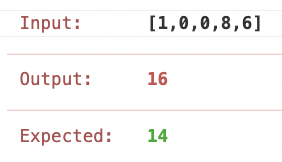# leetcode: 462. Minimum Moves to Equal Array Elements II

kldaji·2022년 7월 1일
0

## leetcode

목록 보기
37/42

### use averageclass Solution {
fun minMoves2(nums: IntArray): Int {
val average = nums.sum() / nums.size
nums.forEach { num ->
}
}
}

### use median

class Solution {
fun minMoves2(nums: IntArray): Int {
nums.sort()
val size = nums.size
val mid = if (size.and(1) == 1) nums[size / 2] else (nums[size / 2] + nums[size / 2 - 1]) / 2

nums.forEach { num ->
}
}
}

### reduce the problem n -> n-2 -> n-4 -> ...

• we don't need specific meeting point, just calculate (max - min) of array.
• let's consider max is 9, and min is 1.
• if we pick the meeting point is 1, the amount of move count will be 8.
• if we pick 2, it will be also 8, and so on.
• so, we just consider max and min value in array then, reduce the array size using two pointers.
• if so, why these approach is same to get the median value?
• let's assume that we pick the meeting point to median value.
• now we can get the amount of move count to (max - median) + (min - median).
• if the size of array is odd, median value does not need to move anymore.
• therefore, pick the meeting value to median value can be the answer of this problem.
class Solution {
fun minMoves2(nums: IntArray): Int {
nums.sort()
var left = 0
var right = nums.size - 1
}